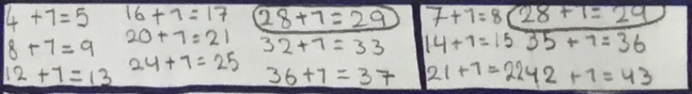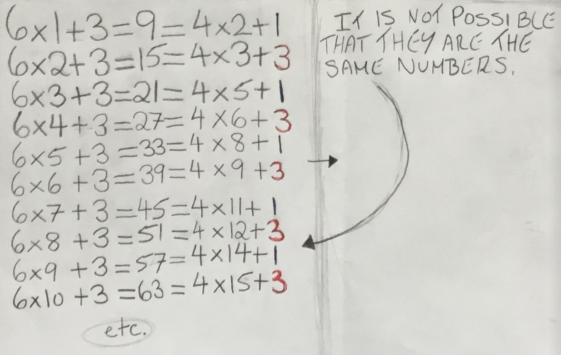You may also likeExploring Wild & Wonderful Number Patterns

EWWNP means Exploring Wild and Wonderful Number Patterns Created by Yourself! Investigate what happens if we create number patterns using some simple rules.Sending Cards

This challenge asks you to investigate the total number of cards that would be sent if four children send one to all three others. How many would be sent if there were five children? Six?Dice and Spinner Numbers

If you had any number of ordinary dice, what are the possible ways of making their totals 6? What would the product of the dice be each time?

Remainders

Age 7 to 14Challenge Level

My number is both a multiple of 5 and a multiple of 6.
Heleen, Luna and Elise from International School of Brussels in Belgium, Christie and Hannah from Peak School in Hong Kong, Hugh from Australia, Zain from Kings Al Barsha in UAE and Laiba from Uphall Primary School in the UK found possible numbers. This is Christie and Hannah's answer:

The number could be thirty, because five and six are both [factors of] thirty. Another answer it could be is sixty or anything that is a multiple of thirty. A rule that we found was that the answer had to end in a zero because there is no multiple of six that ends in a five, and multiples of five have to end in a five or zero. So we knew that the answer had to end in a zero.

Sofia from International School of Brussels used a different method:
It was easy to find this because I just had to multiply 6$\times$5 or 5$\times$6 and I know it's a multiple of both.

Anh Minh from British Vietnamese International School Hanoi in Vietnam used a similar method:
6 = 3$\times$2, so the number must be a multiple of 2, 3 and 5 if it wants to be a multiple of 6 and 5. It must be a number with the one's digit of 0 and be a multiple of 2 and 3. So, the smallest number possible to be multiple of 6 and 5 is 30

Christian from Dulwich College Seoul in South Korea, Shriya from International School Frankfurt in Germany, Majid from Little Ilford School, Aishwarya from Hymers College Junior School, Sam from Banwell Primary School and Justin from Uphall Primary School, all in the UK, used the lowest common multiple. This is Justin's work:
Multiples of 5: 5, 10, 15, 20, 25, 30, 35
Multiples of 6: 6, 12, 18, 24, 30, 36
The first common multiple is 30.
You can work out other answers by multiplying 30 [by] any number.
For example: 30$\times$4=120
120 can be divided by 6 and 5.
The smallest answer will be 30 because there is no other number, that is in the 30 times tables, that is smaller than 30.

My number is a multiple of 4, 5 and 6.
Trafalgar Square Class from Merton Park Primary School in the UK used the number sieve:

We found three numbers on the grid that are multiple of 4, 5 and 6.
They were 60, 120 and 180.

We explored the patterns and started with the multiples of 5 as this was the easiest. We were almost tricked by 160 but we had to recall our times tables to help us.

We predict 240 would be the next one.

Hannah and Christie used the same method they had used before:
The number could be sixty. Another answer it could be is one hundred and twenty or any multiple of sixty and above. The rule is that the number can only end in a zero because four and six do not have any multiple that ends in a five.

Sofia and Sam used Sofia's method. Sam said:
I did 4$\times$5$\times$6=120. Because I know my times tables, I worked out that 60 would also work as an answer. I quickly worked out that 60 was the lowest number possible to get that fitted the criteria. I noticed that all the multiples of 60 worked as well e.g. 120,180, 240

Jerlin from Saltash.net Community School in the UK, Majid, Aishwarya and Christian used listing strategies to find common multiples. Jerlin said:
The smallest number is 60. I first used the number sieve and then tried to work it out without the number sieve. This is what I did -

I wrote down the multiples of 4, 5 and 6. Then I highlighted the common multiples. From the highlighted numbers, I found that the Lowest Common Multiple (LCM) was 60.

I'm thinking of a number that is 1 more than a multiple of 7.
My friend is thinking of a number that is 1 more than a multiple of 4.

Elise used a listing strategy:They are both thinking of 29.

Majid used lowest common multiples again:
They might think of the same number as the original answer, (final answer - 1), is a common multiple of 7 and 4. For example, 28, is a multiple of 7 and 4. Plus 1 is 29. So the friend thinks of 28 + 1 as [one more than] a multiple of 4, and the other person 28 + 1 as [one more than] a multiple of 7, but still providing the same answer.

Ayla from Woodlea Primary School in the UK also sent in 29, Heleen, Luna and Shriya sent in a list of possible numbers, shown below. How are Shriya's numbers related to Majid's answer of 29?
Yes, you could have been thinking of the same number because 4Ã—7=28. If you had been thinking of the same number, your number could have been 29, 57, 85, 113, or 141.

Aishwarya created algebraic expressions for the two numbers:
Using algebra, we can form two expressions for these numbers:
For one more than a multiple of 7 we get $7x+1$, where $x$ is a whole number.
For one more than a multiple of 4 we get $4x + 1$, where $x$ is a whole number.
If we want both these people to be thinking of the same number, we can substitute 4 and 7 into the two expressions separately because they have the same constant of 1 added to them: substituting $x = 4$ into the first expression which would then equal $7(4) + 1 = 29$ and $x = 7$ into the second expression which gives $4(7) + 1 = 29 .$ Therefore $29$ is the smallest same number the two people could be thinking of.
Note: usually mathematicians don't use the same letter twice in the same piece of working, because it is confusing to say that $x=4$ in one expression but $x=7$ in the other. It would be clearer to use two different letters, for example $7x+1$ and $4y+1$, where $x$ and $y$ are whole numbers.

If we look at all the 180 numbers in the number sieve, the numbers which could both could be thinking of which are the same are 57, 85, 113, 141 and 169.

I'm thinking of a number that is 3 more than a multiple of 5.
My friend is thinking of a number that is 8 more than a multiple of 10.

Elise and Luna used a listing strategy. Here is Elise's work:On this problem there are multiple numbers in common because 5 is a [factor] of 10.

They could still be thinking of the same number.

Ayla and Shriya sent in some possible numbers. Aishwarya and Shriya described the possible numbers. Aishwarya said:
8 more than a multiple of 10 is the same as 3 more than a multiple of 5...
Shriya said:
... because 8=5+3. If your numbers were the same, you number could have been any number that ends with eight, except for 8.

I'm thinking of a number that is 3 more than a multiple of 6.
My friend is thinking of a number that is 2 more than a multiple of 4.

Elise and Luna listed numbers again, and Luna spotted a pattern in the list:Ayla, Aishwarya and Shriya all said no. This is Aishwarya's explanation:
No, the two people can never be thinking of the same number. This is because if we look at the sequence of the last digits of the sequence of numbers which are three more than a multiple of 6 we get 9, 5, 1, 7, 3 and this repeats. The last digits of the sequence of numbers which are two more than a multiple of 4 are 6, 0, 4, 8, 2. The last digits for 3 more than a multiple of 6 are always odd whereas the last digit for 2 more than a multiple of 4 are always even. Because of this, they can never think of the same number.

Shriya's explanation was similar:
No, you can not have been thinking of the same number because 3 more than a multiple of 6 is always odd and 2 more than a multiple of 4 is always even.

Can you find a number with the property that when it is divided by each of the numbers 2 to 10, the remainder is always one less than the number it is has been divided by?
Aishwarya found one by searching the multiples of 9:

Firstly, for this number to produce a remainder of 9 when divided by 10, the end digit must be 9. Having figured this out, I decided to look at the multiples of 9, in particular ones in the sequence 81,171, 261, 351 ”¦ . (multiples of 9 ending in 1, so that when 8 is added, the last digit will be 9)

Then I would test whether one more than these numbers was a multiple of 8, if so then I'd test whether one more than the resulting number was a multiple of 7. The first number that caused this was 2511 : 2511$\div$9 = 279, 2512$\div$8 = 314, 2513$\div$7 = 359... .

Therefore the smallest number that satisfies the above conditions is 2510 + 9 = 2519

Shriya generated a long list of conditions and a careful logical argument to make the searching quicker. Click here to see Shriya's work.

Louie from St John's C of E Primary School, Abram in the UK sent in a neat solution. Louie used some of this reasoning, but in a different order:
59 is 4 more than a multiple of 5, so it is 1 less than the next multiple of 5 (60)
59 is 3 more than a multiple of 4, so it is 1 less than the next multiple of 4 (60)
59 is 2 more than a multiple of 3, so it is 1 less than the next multiple of 3...
and so on
We want a number that is 9 more than a multiple of 10, and so it will be 1 less than the next multiple of 10.
It will be 8 more than a multiple of 9, so 1 less than the next multiple of 9...
and so on

Louie wrote:

Let's say unknown number is $n$

When $\dfrac n{10}$ remainder is $9$ - ends in a $9$

When $\dfrac n{9}$ remainder is $8$ - multiple of $90 - 1$

When $\dfrac n{8}$ remainder is $7$

The lowest common multiple of $90$ & $8$ is $360.$  $360$ is a multiple of $2, 3, 4, 5, 6$ so ignore them.

$360\times7 = 2520$
$2520-1=2519$